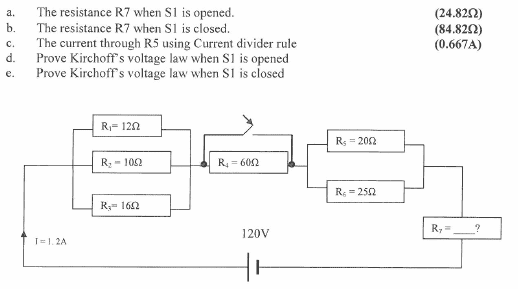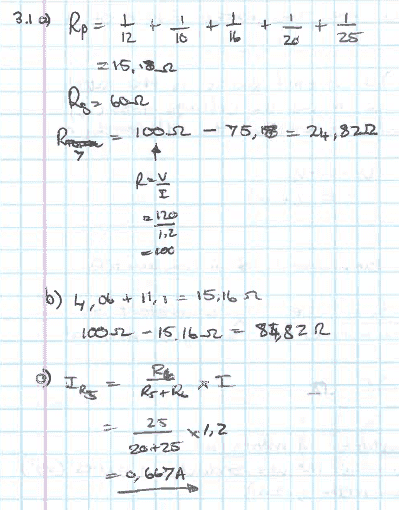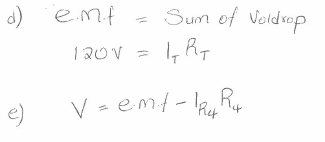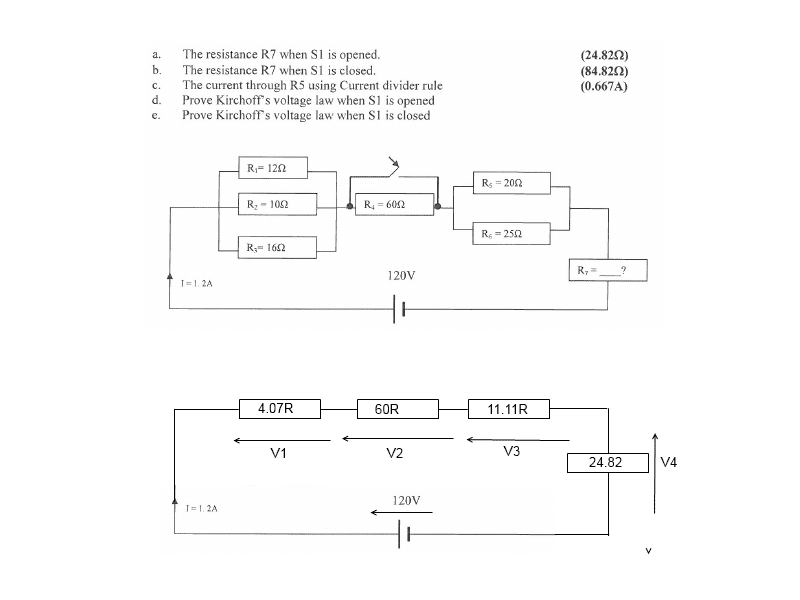# Kirchhoff's Law Problem -- Resistors in Series and Parallel and a Switch

• DevonZA
In summary, the conversation is about a circuit diagram and the use of Kirchhoff's Voltage Law (KVL) to calculate the total resistance of parallel resistors and the voltage drops around a loop. There is also discussion about a specific device, R7, and questions about the source of the problem and its author. The conversation ends with a clear explanation of KVL and its application in the circuit.

## Homework Statement## Homework Equations

Rparellel=1/R1+1/R2...
IR1=R2/R1+R2 x I

## The Attempt at a SolutionI am unsure of how to answer d) and e) using KVL because I count 4 junctions?
Where should I start?

Check your first equation ! It's not for Rpalrallel but for 1/=Rpalrallel !

And the 20 and 25 Ohm resistors are NOT parallel to e.g. the 12 Ohm !

Yes sorry that was a typo.
The total resistance for the parallel resistors still equals 15.18ohms but yes I should have done them separately:
(1/12+1/10+1/16)^-1 = 4.07ohms
(1/20+1/25)^-1= 11.11ohms

Do my values look correct?

I only know $${1\over 12} + {1\over 10}+ {1\over 16}+ {1\over 20}+ {1\over 25} \ne 15.82$$

(1/12+1/10+1/16+1/20+1/25)^-1=15.18 ohms

Yes values are answers in the book

Then why ask if the values are correct ?

DevonZA said:
(1/12+1/10+1/16+1/20+1/25)^-1=15.18 ohms
No. $${1\over 12} + {1\over 10}+ {1\over 16}+ {1\over 20}+ {1\over 25} = {1\over 2.98}$$However,$${1\over {1\over 12} + {1\over 10}+ {1\over 16} } + {1 \over {1\over 20}+ {1\over 25} } = 4.07 + 11.11 = 15.18$$

Last edited:DevonZA said:

## Homework Statement

[ ATTACH=full]99511[/ATTACH]What sort of device is R7 that its resistance changes depending on whether the switch is opened or closed

The parts d and e are a bit weird, because basically you use KVL to calculate R7 in a and b.
And it wouldn't be proof, just showing.
I should think they want you to show that 1.2 A * (4.07 + 60 + 11.11 + 24.82) Ohm = 120 V

•DevonZA
+1

Although I prefer to make the voltages around the loop sum to zero.

•SammyS
@DevonZA ,

Are you saying that this problem is from a textbook?

What book? Who is the author ?

Last edited:
CWatters said:
+1

Although I prefer to make the voltages around the loop sum to zero.
Can you show this?

SammyS said:
@DevonZA ,

Are you saying that this problem is from a textbook?

What book? Who is the author ?
Hi Sammy this is from our varsity study guide. The author is most likely the lecturer who doesn't respond to me hence why I must ask these questions on here.

CWatters said:
Although I prefer to make the voltages around the loop sum to zero.
DevonZA said:
Can you show this?

With reference to the equivalent circuit below... KVL says that going around a loop the voltages sum to zero.If I arbitrarily choose to start at the -ve terminal of the battery and go around clockwise we have to prove that..

+120 + (-V1) + (-V2) + (-V3) + (-V4) = 0

V1 = 1.2 * 4.07 = 4.884V
V2 = 1.2 * 60 = 72V
V3 = 1.2 * 11.11 = 13.332V
V4 = 1.2 * 24.82 = 29.784V

Substitute..

+120 - 4.884 - 72 -13.332 - 29.784 = 0

•DevonZA
CWatters said:
With reference to the equivalent circuit below... KVL says that going around a loop the voltages sum to zero.
View attachment 99596

If I arbitrarily choose to start at the -ve terminal of the battery and go around clockwise we have to prove that..

+120 + (-V1) + (-V2) + (-V3) + (-V4) = 0

V1 = 1.2 * 4.07 = 4.884V
V2 = 1.2 * 60 = 72V
V3 = 1.2 * 11.11 = 13.332V
V4 = 1.2 * 24.82 = 29.784V

Substitute..

+120 - 4.884 - 72 -13.332 - 29.784 = 0
Thank you for the nice clear explanation CWatters